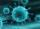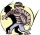# Population

What is the population of the city with 3% annual growth, if in 10 years the city will have 60,000 residents?

Result

n =  44646

#### Solution:Leave us a comment of example and its solution (i.e. if it is still somewhat unclear...):Be the first to comment!## Next similar examples:

1. VirusWe have a virus that lives one hour. Every half hour produce two child viruses. What will be the living population of the virus after 3.5 hours?
2. PopulationThe town has 65,000 inhabitants. 40 years ago there were 157,000. How many people will live in town in 10 years if the average rate in population is as in previous years?
3. Theorem proveWe want to prove the sentence: If the natural number n is divisible by six, then n is divisible by three. From what assumption we started?
4. A perineumA perineum string is 10% shorter than its original string. The first string is 24, what is the 9th string or term?
5. The crimeThe crime rate of a certain city is increasing by exactly 7% each year. If there were 600 crimes in the year 1990 and the crime rate remains constant each year, determine the approximate number of crimes in the year 2025.
6. Geometric progression 2There is geometric sequence with a1=5.7 and quotient q=-2.5. Calculate a17.
7. BankPaul put 10000 in the bank for 6 years. Calculate how much you will have in the bank if he not pick earned interest or change deposit conditions. The annual interest rate is 3.5%, and the tax on interest is 10%.
8. Six termsFind the first six terms of the sequence a1 = -3, an = 2 * an-1
9. Sequence - 5 membersWrite first five members of the sequence ?
10. SequenceFind the common ratio of the sequence -3, -1.5, -0.75, -0.375, -0.1875. Ratio write as decimal number rounded to tenth.
11. GP membersThe geometric sequence has 10 members. The last two members are 2 and -1. Which member is -1/16?
12. GP - 8 itemsDetermine the first eight members of a geometric progression if a9=512, q=2
13. LogarithmDetermine the number whose decimal logarithm is -3.8.
14. Geometric progression 48,4√2,4,2√2
15. Geometric sequence 4It is given geometric sequence a3 = 7 and a12 = 3. Calculate s23 (= sum of the first 23 members of the sequence).
16. Five membersWrite first 5 members geometric sequence and determine whether it is increasing or decreasing: a1 = 3 q = -2
17. Tenth memberCalculate the tenth member of geometric sequence when given: a1=1/2 and q=2